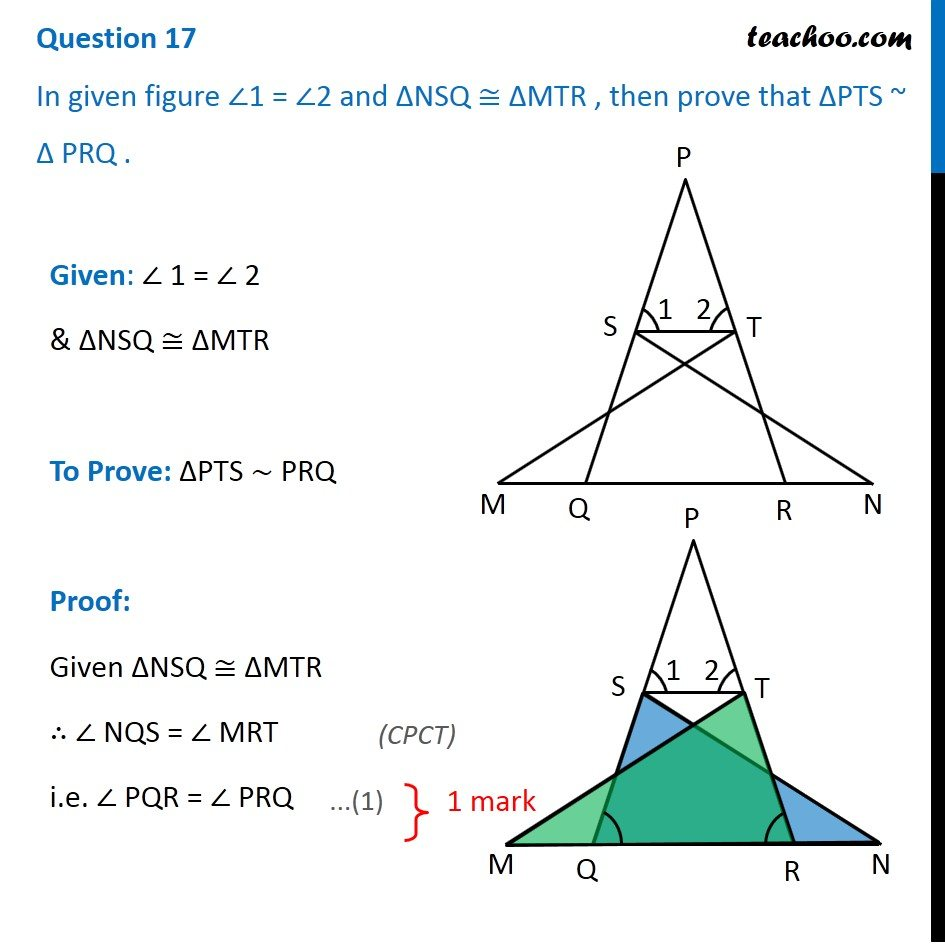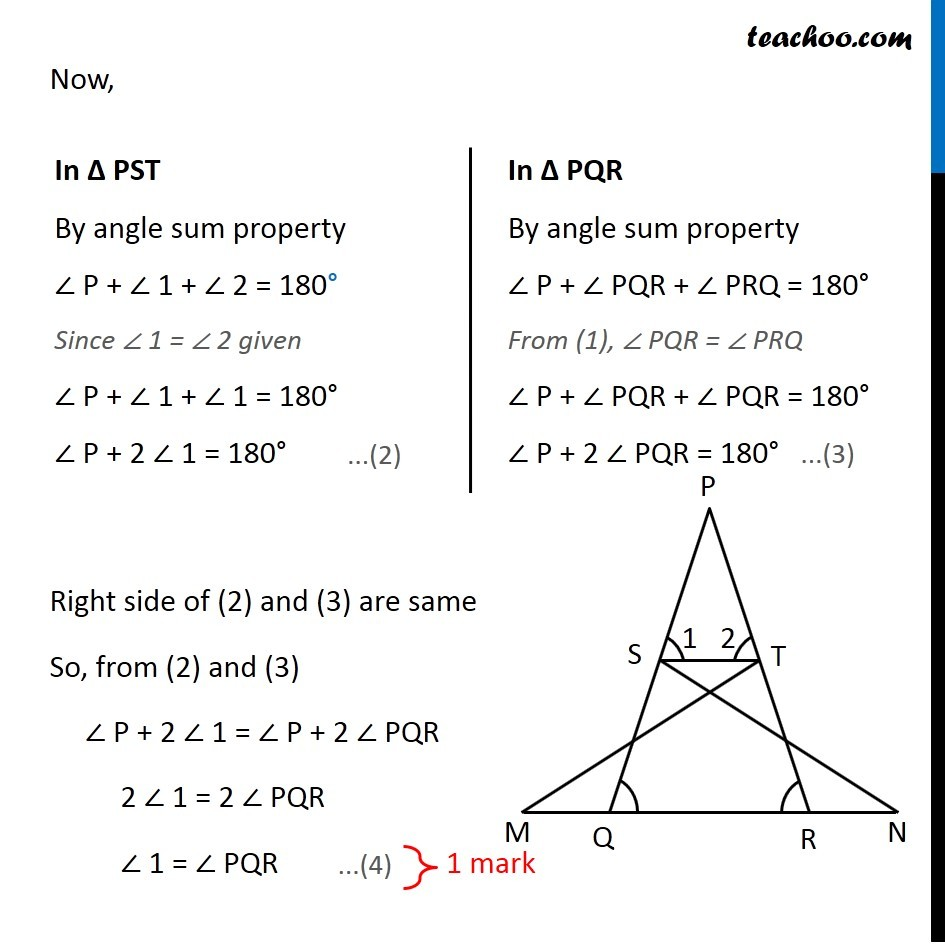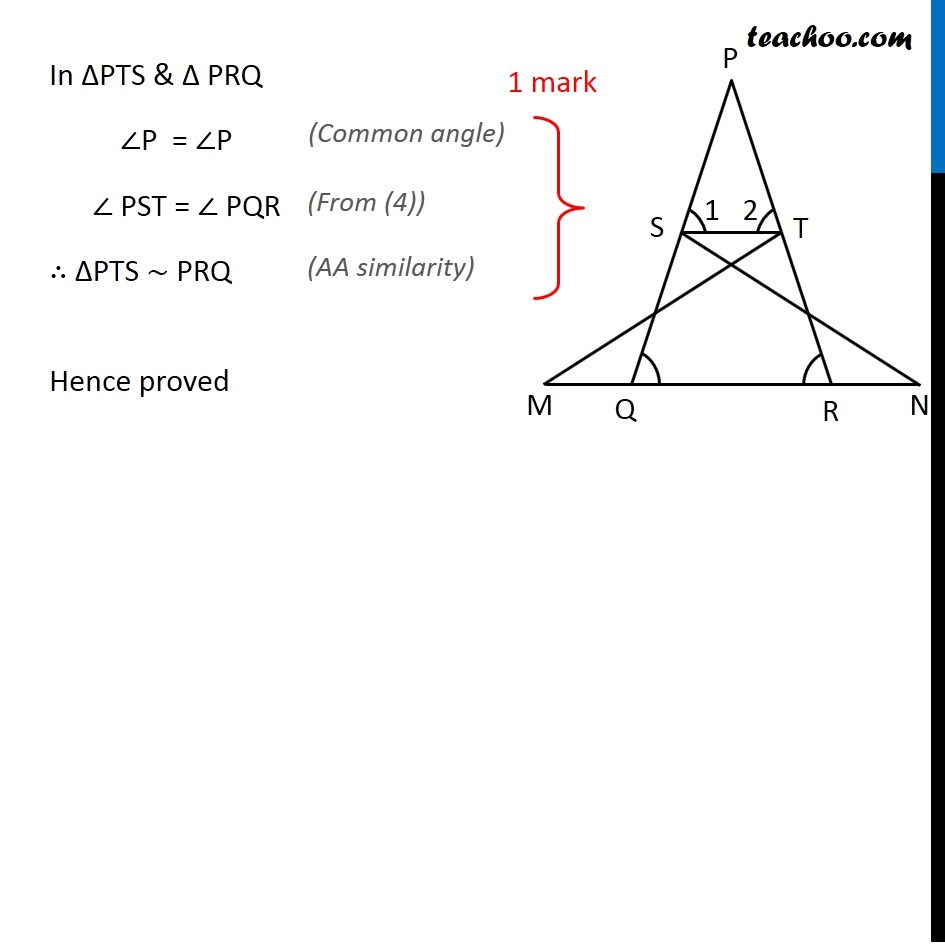CBSE Class 10 Sample Paper for 2018 Boards

Class 10
Solutions of Sample Papers for Class 10 Boards

### In an equilateral triangle ABC, D is a point on the side BC such that BD = 1/3 BC. Prove that 9AD 2 = 7AB 2

This is a question of CBSE Sample Paper - Class 10 - 2017/18.### In an equilateral triangle ABC, D is a point on the side BC such that BD = 1/3 BC. Prove that 9AD 2 = 7AB 2

This is exactly same as Ex 6.5, 15.

### Transcript

Question 17 In given figure ∠1 = ∠2 and ∆NSQ ≅ ∆MTR , then prove that ∆PTS ~ ∆ PRQ . Given: ∠ 1 = ∠ 2 & ΔNSQ ≅ ΔMTR To Prove: ΔPTS ∼ PRQ Proof: Given ΔNSQ ≅ ΔMTR ∴ ∠ NQS = ∠ MRT i.e. ∠ PQR = ∠ PRQ Now, In Δ PST By angle sum property ∠ P + ∠ 1 + ∠ 2 = 180° Since ∠ 1 = ∠ 2 given ∠ P + ∠ 1 + ∠ 1 = 180° ∠ P + 2 ∠ 1 = 180° In Δ PQR By angle sum property ∠ P + ∠ PQR + ∠ PRQ = 180° From (1), ∠ PQR = ∠ PRQ ∠ P + ∠ PQR + ∠ PQR = 180° ∠ P + 2 ∠ PQR = 180° Right side of (2) and (3) are same So, from (2) and (3) ∠ P + 2 ∠ 1 = ∠ P + 2 ∠ PQR 2 ∠ 1 = 2 ∠ PQR ∠ 1 = ∠ PQR In ΔPTS & Δ PRQ ∠P = ∠P (Common angle) ∠ PST = ∠ PQR (From (4)) ∴ ΔPTS ∼ PRQ (AA similarity) Hence proved